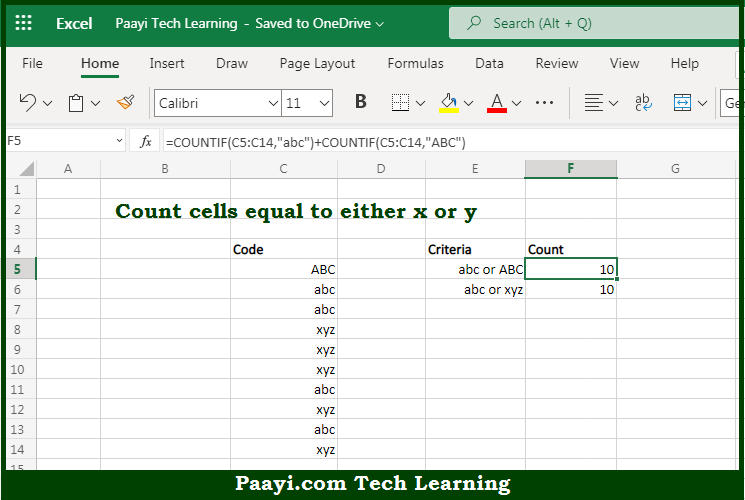# Learn How to Count Cells Equal to Either x or y

Written by | 0 Comments | 503 Views

In this article, you will learn how to use the Microsoft Excel COUNT formula and its purpose in Microsoft Excel. You will also get to know how to count cells equal to either x or y and see the generic formula.

Count Cells Equal to Either x or y Using Microsoft Excel Count Formula

The main purpose of the Microsoft Excel COUNT formula is to count the number of cells equal to either one value OR another. Here we will learn how to count cells equal to either x or y.  That implies, with the help of the COUNT formula you can able to count the number of cells equal to either one value OR another, you use a formula that uses the COUNTIF function twice. So, with the help of the COUNT formula, you can able to count the number of cells equal to either one value OR another.

General Syntax of the COUNT Count Cells Equal to Either x or y

=COUNTIF(rng,value1) + COUNTIF(rng,value2)

The Explanation for the Count Cells Equal to Either x or ySo we know that Microsoft Excel COUNT formula you can able to count the number of cells equal to either one value OR another. Here we will learn how to count cells equal to either x or y. COUNTIF counts the number of cells in a range that matches supplied criteria. In the example given above, the criteria for the first COUNTIF is "apples" and the criteria for the second COUNTIF is "pears".

It should be noted that COUNTIF is not case-sensitive. So, with the help of the COUNT formula, you can able to count the number of cells equal to either one value OR another.